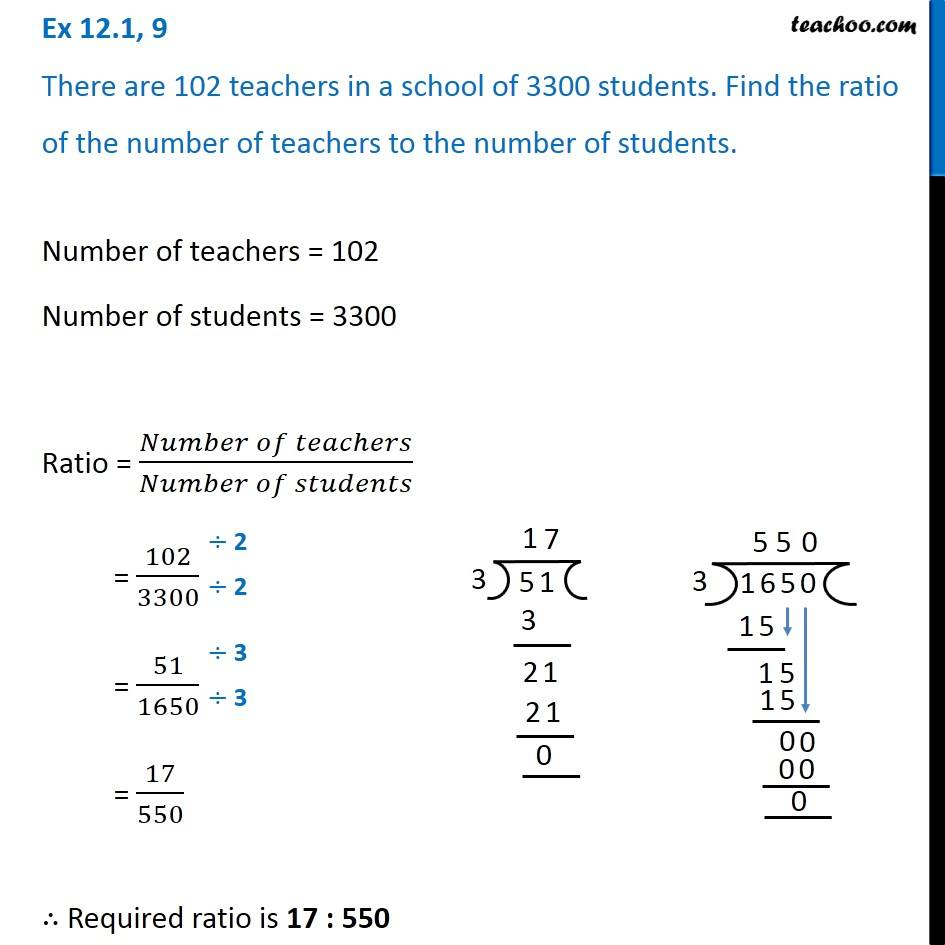Ratios

Chapter 12 Class 6 Ratio and Proportion
Concept wiseLearn in your speed, with individual attention - Teachoo Maths 1-on-1 Class

### Transcript

Ex 12.1, 9 There are 102 teachers in a school of 3300 students. Find the ratio of the number of teachers to the number of students.Number of teachers = 102 Number of students = 3300 Ratio = (𝑁𝑢𝑚𝑏𝑒𝑟 𝑜𝑓 𝑡𝑒𝑎𝑐ℎ𝑒𝑟𝑠)/(𝑁𝑢𝑚𝑏𝑒𝑟 𝑜𝑓 𝑠𝑡𝑢𝑑𝑒𝑛𝑡𝑠) = 102/3300 = 51/1650 = 17/550 ∴ Required ratio is 17 : 550